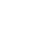• Descriptive Statistics: Summarizes and describes characteristics of a set of scores for a group
• Frequency Distribution: # of people who received each score
• Histogram: frequency distribution turned into a graph.
• The measure of central tendency: mean (average), median (middle), mode (most often)
• The measure of variability: provide info about the spread of scores (i.e range) (highest- lowest) least informative

Creating Deviate Score

Variance: distance between each store.

• Find the mean of all scores (M)
• Find how much each value deviates from that (X)
• Square all those values, making them all positive and ascertain a value (x^2) [Variance]
• Divide Variance by mean, then square root it to get the standard deviation

Normal Curve: symmetrical bell-shape curve that represent distribution in theory of the population.

SD can divide the curve into segments (68% of population fall within SD: -1-+1) (95% between SD: -2-+2 2% are above it) and nearly 100% between Sd: -3-+3 0.1% of being higher)

Based on the % of each segment allows us to predict the probability of an event transpiring. (through addition)

Psychologists TRY TO PREDICT WHY THESE EVENTS TRANSPIRE AND EXPLAIN THEM

Total variance= (variance account for by changing variable + variance not accounted for (random: error variance))

Applies to experiments and correlational studies

Pearson product-moment correlation coefficient: reflects direction/ strength of relation between two variables.

.5 coefficient DOES NOT mean that variables A and B and 50 percent related. SQUARE the r value to get the amount of variance that the two variables share or have in common. (.5^2=.25) 25% of A can account 25% of the data variance in B. REMEMBER CORRELATION IS NOT CAUSATION

If predicator variable is closest related to criterion variable then accurate prediction can be made

Factor Analysis: Take a large amount of correlations and makes small cluster, each containing highly-related correlations (uses computers

Can identify correlations between variables in a correlation matrix but can’t explain what those relations mean. Psychologists must do the rest.

Things that influence statistics:

1) Size of the difference (Big difference= more significant)

2) Variability of measure (More variability= less significant)

3) # of measurements (More measurement= more significance)

Inferential statistics: how confident are we in drawing conclusions/ inferences about population based on sample

The finding must be Statistically significant, meaning it didn’t happen by chance and was based on facts. Performing test multiple times will allow for a more accurate distribution

Null Hypothesis: any difference between sample data is due to chance

Cite this article as: William Anderson (Schoolworkhelper Editorial Team), "Statistics in Psychology: Variance, Deviation," in SchoolWorkHelper, 2021, https://schoolworkhelper.net/statistics-in-psychology-variance-deviation/.